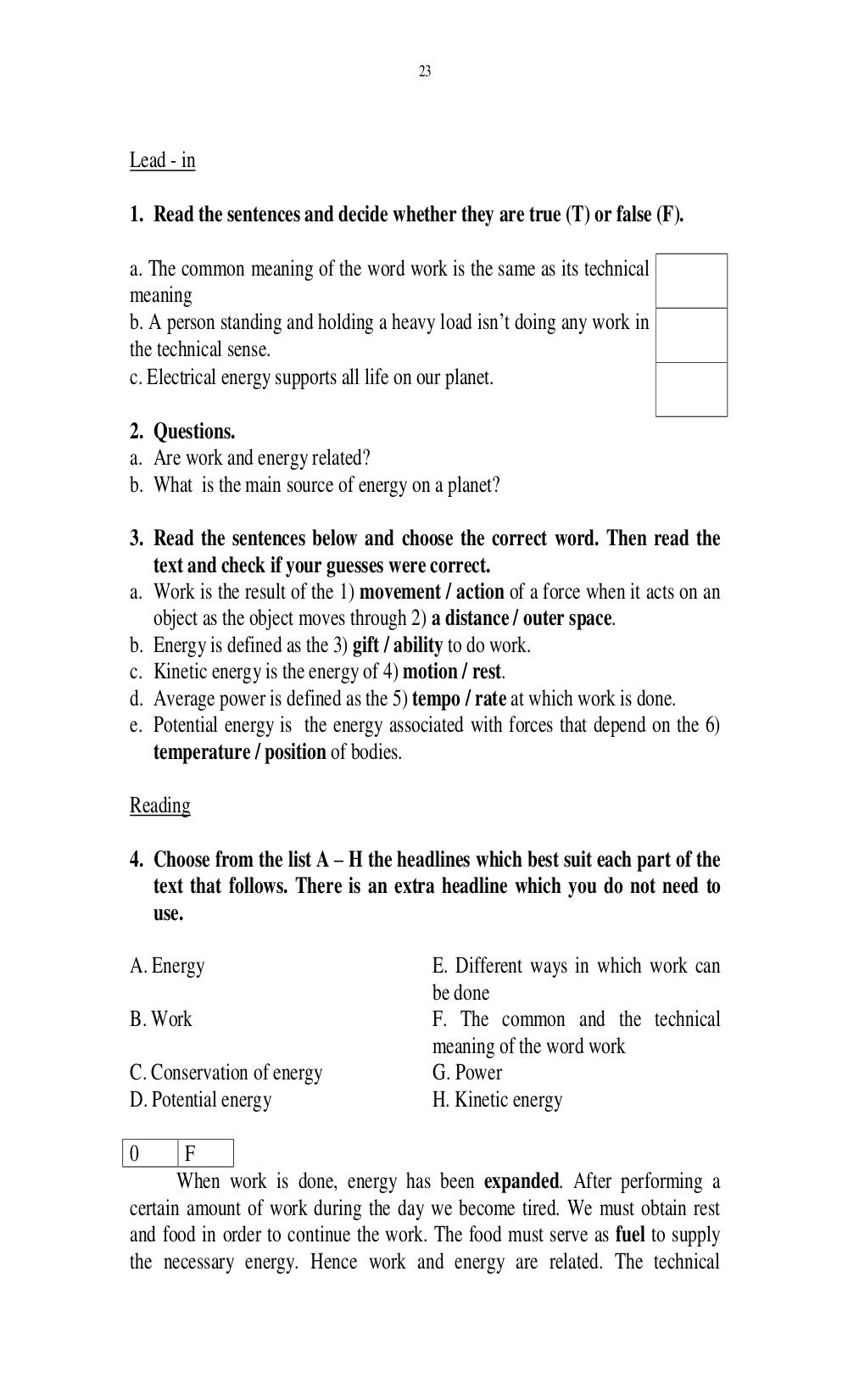# Английский язык. Ильичева Н.А - 23 стр.

Составители:

Рубрика:

• ## Иностранный язык23
1. Read the sentences and decide whether they are true (T) or false (F).
a. The common meaning of the word work is the same as its technical
meaning
b. A person standing and holding a heavy load isnt doing any work in
the technical sense.
c. Electrical energy supports all life on our planet.
2. Questions.
a. Are work and energy related?
b. What is the main source of energy on a planet?
3. Read the sentences below and choose the correct word. Then read the
text and check if your guesses were correct.
a. Work is the result of the 1) movement / action of a force when it acts on an
object as the object moves through 2) a distance / outer space.
b. Energy is defined as the 3) gift / ability to do work.
c. Kinetic energy is the energy of 4) motion / rest.
d. Average power is defined as the 5) tempo / rate at which work is done.
e. Potential energy is the energy associated with forces that depend on the 6)
temperature / position of bodies.
4. Choose from the list A H the headlines which best suit each part of the
text that follows. There is an extra headline which you do not need to
use.
A. Energy E. Different ways in which work can
be done
B. Work F. The common and the technical
meaning of the word work
C. Conservation of energy G. Power
D. Potential energy H. Kinetic energy
0 F
When work is done, energy has been expanded. After performing a
certain amount of work during the day we become tired. We must obtain rest
and food in order to continue the work. The food must serve as fuel to supply
the necessary energy. Hence work and energy are related. The technical23

1. Read the sentences and decide whether they are true (T) or false (F).

a. The common meaning of the word work is the same as its technical
meaning
b. A person standing and holding a heavy load isn’t doing any work in
the technical sense.
c. Electrical energy supports all life on our planet.

2. Questions.
a. Are work and energy related?
b. What is the main source of energy on a planet?

3. Read the sentences below and choose the correct word. Then read the
text and check if your guesses were correct.
a. Work is the result of the 1) movement / action of a force when it acts on an
object as the object moves through 2) a distance / outer space.
b. Energy is defined as the 3) gift / ability to do work.
c. Kinetic energy is the energy of 4) motion / rest.
d. Average power is defined as the 5) tempo / rate at which work is done.
e. Potential energy is the energy associated with forces that depend on the 6)
temperature / position of bodies.

4. Choose from the list A – H the headlines which best suit each part of the
text that follows. There is an extra headline which you do not need to
use.

A. Energy                                  E. Different ways in which work can
be done
B. Work                                    F. The common and the technical
meaning of the word work
C. Conservation of energy                  G. Power
D. Potential energy                        H. Kinetic energy

0       F
When work is done, energy has been expanded. After performing a
certain amount of work during the day we become tired. We must obtain rest
and food in order to continue the work. The food must serve as fuel to supply
the necessary energy. Hence work and energy are related. The technical